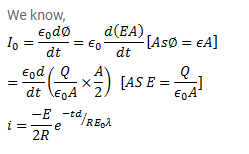# A parallel-plate capacitor having plate-area A

Question:

A parallel-plate capacitor having plate-area $\mathrm{A}$ and plate separation $\mathrm{d}$ is joined to a battery of emf $\epsilon$ and internal resistance $\mathrm{R}$ at $\mathrm{t}=0$. Consider a plane surface of area $\mathrm{A} / 2$, parallel to the plates and situated symmetrically between them. Find the displacement current through this surface as a function of time.

Solution: